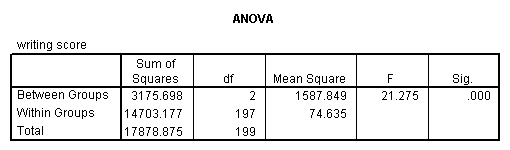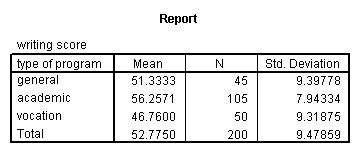## One-way ANOVA

A one-way analysis of variance (ANOVA) is used when you have a categorical independent variable (with two or more categories) and a normally distributed interval dependent variable and you wish to test for differences in the means of the dependent variable broken down by the levels of the independent variable. For example, using the hsb2 data file, say we wish to test whether the mean of write differs between the three program types (prog). The command for this test would be:

oneway write by progThe mean of the dependent variable differs significantly among the levels of program type. However, we do not know if the difference is between only two of the levels or all three of the levels. (The F test for the Model is the same as the F test for prog because prog was the only variable entered into the model. If other variables had also been entered, the F test for the Model would have been different from prog.) To see the mean of write for each level of program type,

means tables = write by prog.From this we can see that the students in the academic program have the highest mean writing score, while students in the vocational program have the lowest.